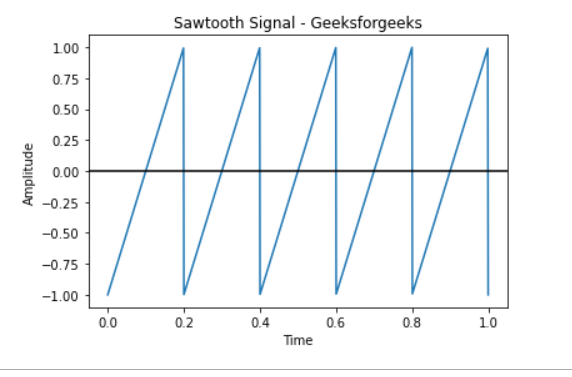# Plotting a Sawtooth Wave using Matplotlib

• Last Updated : 03 Jan, 2021

Prerequisites:

A sawtooth waveform is a non-sinusoidal waveform because its teeth look like a saw. In an inverse (or reverse) sawtooth waveform the wave suddenly ramps downwards and then rises sharply. With Matplotlib we can draw different types of Graphical data. In this article, we will try to understand, How can we plot sawtooth waves using the Scipy python module.

### Approach:

• Import required module.
• Create a sample rate. The NumPy linspace function is a tool in Python for creating numeric sequences that return evenly spaced numbers over a specified interval.

Syntax:

numpy.linspace(start, stop, num = 50,
endpoint = True, retstep = False, dtype = None)

• Plot a sawtooth wave.
• Label the graph.
• Display Graph.

### Function

Syntax:

scipy.signal.sawtooth(t)

Parameter:

• t: The input time array.

Return:

Output array containing the sawtooth waveform.

Program:

## Python3

 `from` `scipy ``import` `signal``import` `matplotlib.pyplot as plot``import` `numpy as np`` ` `t ``=` `np.linspace(``0``, ``1``, ``1000``, endpoint``=``True``)`` ` `# Plot the sawtooth wave``plot.plot(t, signal.sawtooth(``2` `*` `np.pi ``*` `5` `*` `t))`` ` `# Give x, y, title axis label``plot.xlabel(``'Time'``)``plot.ylabel(``'Amplitude'``)``plot.title(``'Sawtooth Signal - Geeksforgeeks'``)`` ` `plot.axhline(y``=``0``, color``=``'k'``)`` ` `# Display``plot.show()`

Output:My Personal Notes arrow_drop_up